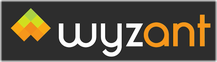HTML5 Apps Desmos Graphing Calculator Free Web browser tool that is great at graphing multiple precalculus equations on a grid. iPad Apps Apple's App Store MathGraphing Calculator Best Graphing CalculatorWolframAlpha The Google of MathematicsMath Ref Best Math and Physics FormulasCalcChat Solutions to Ron Larson Calculus Textbook ProblemsFormulas - Formulas for Calculus More FormulasPCalc Best CalculatorSymbolic Calculator HD Symbolic Calculator (CAS)PocketCAS Pro Symbolic Calculator (CAS)SpaceTime GraphingPi Cubed CalculatorStatistics 1 Statistics PhysicsiScience Mathematics, Physics and Chemistry FormulasConvert ~the unit calculator The Best Unit ConverterG Gravity Simulator - Force and AccelerationCradle Newtons Cradle - Conservation of MomentumIons Charge Simulator - Point Charges and ForcePhysics Bites! - Lenses Optics Simulator - Lenses and Focal Points SAT / ACT MathSAT QOTD The Official SAT Question of the Day, The College BoardMath Ref Math and Physics FormulasTimer Timed Test AidChronology - Timer Timed Test Aid ChessChess With Friends Play chess with your friends wirelessly on your own timet Chess Pro Get suggested moves from a chess masterLearn Chess Free Chess 101 ManualChess Problems Real Professional tournament 2 and 3 move checkmate puzzlesWarChess Battle Chess. Animated kills. Kids love this.KHET 2X Khet or Laser Chess